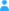Sign in

|

# Parameter square_​of_​lagrangian_​tendency_​of_​air_​pressure

## Properties

Name square_of_lagrangian_tendency_of_air_pressure "square_of_X" means X*X. "tendency_of_X" means derivative of X with respect to time. The Lagrangian tendency of a quantity is its rate of change following the motion of the fluid, also called the "material derivative" or "convective derivative". The Lagrangian tendency of air pressure, often called "omega", plays the role of the upward component of air velocity when air pressure is being used as the vertical coordinate. If the vertical air velocity is upwards, it is negative when expressed as a tendency of air pressure; downwards is positive. not specified not specified

## Source

The parameter was taken from the NetCDF CF Metadata Convention.

--> </